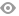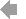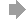﻿ Page 9 - dysertaciyahrynenko
Basic HTML VersionView Full Version
Page 9 - dysertaciyahrynenko
P. 9

``````9

plotting true stress-strain diagrams makes it possible to determine true stresses

near concentrators in structural elements under complex loading. The use of these

diagrams  in  engineering  calculations  and  computer  modelling  using  the  FEM

significantly increases their accuracy. Modelling of the damage  accumulation

was carried out using the energy criterion. The damage parameter is introduced

as the ratio of the elastoplastic strain energy of the local volume to its critical

value. The elastoplastic strain energy of the local volume of the structural element

is calculated using FEM. The critical value of the elastoplastic strain (fracture)

energy is taken as a characteristic of the resistance to fracture of the material under

the  given  conditions  of  load,  temperature,  and  working  environment.  It  is

determined experimentally as the area under the "true stress - true strain" curve,

where  the  true  strains  in  the  local  volume  are  calculated  on  the  basis  of

displacement  data  in  two  directions  on  the  basis  of  0.5  mm  using  the  ODIC

methid. The value of the true stresses in the area of maximum strain is determined

by taking into account the change in the cross-sectional area due to the change in

the diameter of the sample during its deformation by uniaxial tension. The fracture

energy and damage parameter for the modified Bridgman sample made of steel

22K  under  different  load  regimes  were  determined  theoretically  and

experimentally.

The  research  methodology  and  the  mathematical  apparatus  used  in  the

study are substantiated. To solve the formulated scientific problems, the methods

of  the  mechanics  of  a  deformable  solid  were  used,  primarily  the  theory  of

elastoplasticity  and  continuum  damage  mechanics.  Numerical  studies  were

carried out using the finite element method in the environment of the ANSYS

2022R1 software complex, in which three-dimensional computer models were

created.

All  the  theoretical  developments  of  the  thesis  are  brought  to  a  specific

engineering methodology for predicting the safe operation of elements of thermal

power equipment, taking into account the action of the operating environment.``````4   5   6   7   8   9   10   11   12   13   14